How Cheenta works to ensure student success?
Explore the Back-Story

# Test of Mathematics Solution Subjective 124 - Graph sketchingThis is a Test of Mathematics Solution Subjective 124 (from ISI Entrance). The book, Test of Mathematics at 10+2 Level is Published by East West Press. This problem book is indispensable for the preparation of I.S.I. B.Stat and B.Math Entrance.

## Problem

Sketch on plain paper, the graph of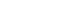## Solution

There are several steps to find graph of a function. We will use calculus to analyze the function. Here y=f(x)

1. Domain: The function is defined at all real numbers except x =1 and x = -1 which makes the denominator 0.
2. Even/Odd: Clearly f(x) = f(-x). Hence it is sufficient to investigate the function for positive values of x and then reflect it about y axis.
3. Critical Points: Next we investigate the critical points. Critical Points are those values of x for which the first derivative of f(x) is either 0 or undefined. Since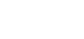, then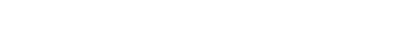.
This implies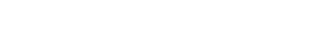Hence critical points are x =0 , 1, -1
4. Monotonicity: The first derivative is negative for all positive values of x (note that we are only investigating for positive x values, since we can then reflect the picture about y axis as previously found). Hence the function is 'decreasing' for all positive value of x.
5. Second Derivative: We compute the second derivative to understand a couple things:
1. convexity/concavity of the function
2. examine whether the critical points are maxima, minima, inflection points.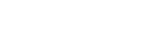Clearly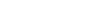implying at x = 0 we have local maxima. Since f(0) = - 1, we have (0, -1) as a local maxima.
Also the second derivative is negative from x = 0 to x = 1 and positive after x = 1. Hence the curve is under-tangent (concave) from x = 0 to x = 1, and above-tangent (convex) from x =1 onward.
6. Vertical Asymptote: We next examine what happens near x = 1. We want to know what happens when we approach x=1 from left and from right. To that end we compute the following limits:
1.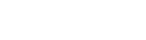(since the denominator gets infinitesimally small with a negative sign, and numerator is about 2)
2.(since the denominator gets infinitesimally small with a positive sign, and numerator is about 2)
7. Horizontal Asymptote: Finally we examine what happens when x approaches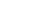. To that end we compute the following: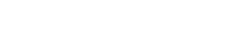8. Drawing the graph:
1. Local Maxima at (0,-1)
2. Even function hence we draw for positive x values and reflect about y axis
3. Vertical asymptote at x =1
4. From x = 0 to 1, the function decreasing to negative infinity, staying under tangent all the time.
5. From x = 1 to positive infinity, the function decreases from positive infinity to 1 staying above tangent all the time.
6. Horizontal Asymptote at y= 1## Chatuspathi:

• What is this topic: Graph Sketching using Calculus
• What are some of the associated concept: Maxima, Minima, Derivative, Convexity, Concavity, Asymptotes
• Where can learn these topics: Cheenta I.S.I. & C.M.I. course, discusses these topics in the 'Calculus' module.
• Book Suggestions: Calculus of One Variable by I.A. Maron, Play with Graph (Arihant Publication)This is a Test of Mathematics Solution Subjective 124 (from ISI Entrance). The book, Test of Mathematics at 10+2 Level is Published by East West Press. This problem book is indispensable for the preparation of I.S.I. B.Stat and B.Math Entrance.

## Problem

Sketch on plain paper, the graph of## Solution

There are several steps to find graph of a function. We will use calculus to analyze the function. Here y=f(x)

1. Domain: The function is defined at all real numbers except x =1 and x = -1 which makes the denominator 0.
2. Even/Odd: Clearly f(x) = f(-x). Hence it is sufficient to investigate the function for positive values of x and then reflect it about y axis.
3. Critical Points: Next we investigate the critical points. Critical Points are those values of x for which the first derivative of f(x) is either 0 or undefined. Since, then.
This impliesHence critical points are x =0 , 1, -1
4. Monotonicity: The first derivative is negative for all positive values of x (note that we are only investigating for positive x values, since we can then reflect the picture about y axis as previously found). Hence the function is 'decreasing' for all positive value of x.
5. Second Derivative: We compute the second derivative to understand a couple things:
1. convexity/concavity of the function
2. examine whether the critical points are maxima, minima, inflection points.Clearlyimplying at x = 0 we have local maxima. Since f(0) = - 1, we have (0, -1) as a local maxima.
Also the second derivative is negative from x = 0 to x = 1 and positive after x = 1. Hence the curve is under-tangent (concave) from x = 0 to x = 1, and above-tangent (convex) from x =1 onward.
6. Vertical Asymptote: We next examine what happens near x = 1. We want to know what happens when we approach x=1 from left and from right. To that end we compute the following limits:
1.(since the denominator gets infinitesimally small with a negative sign, and numerator is about 2)
2.(since the denominator gets infinitesimally small with a positive sign, and numerator is about 2)
7. Horizontal Asymptote: Finally we examine what happens when x approaches. To that end we compute the following:8. Drawing the graph:
1. Local Maxima at (0,-1)
2. Even function hence we draw for positive x values and reflect about y axis
3. Vertical asymptote at x =1
4. From x = 0 to 1, the function decreasing to negative infinity, staying under tangent all the time.
5. From x = 1 to positive infinity, the function decreases from positive infinity to 1 staying above tangent all the time.
6. Horizontal Asymptote at y= 1## Chatuspathi:

• What is this topic: Graph Sketching using Calculus
• What are some of the associated concept: Maxima, Minima, Derivative, Convexity, Concavity, Asymptotes
• Where can learn these topics: Cheenta I.S.I. & C.M.I. course, discusses these topics in the 'Calculus' module.
• Book Suggestions: Calculus of One Variable by I.A. Maron, Play with Graph (Arihant Publication)

### Knowledge Partner